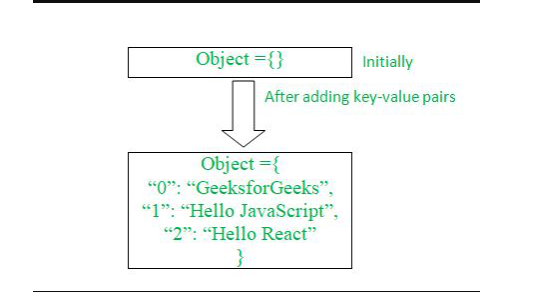Related Articles

# How to create an object from the given key-value pairs using JavaScript ?

• Difficulty Level : Basic
• Last Updated : 28 Jun, 2021

In this article, we will learn to create an object from the given key-value pairs using JavaScript.

Problem Statement: You are given different key-value pair(s), in any form like an array, and using those key-value pairs you need to construct an object which will have those key-value pair(s).

Let us understand with the help of the following pictorial representation.Approach: We first need to declare an empty object, with any suitable name, then by using various JavaScript techniques and methods, we need to insert the given key-value pairs into the previously created empty object.

Let us first try to understand that how we could create an empty object in JavaScript. Creating an empty object in JavaScript could be achieved in several ways.

Example 1:

```let object = {};
console.log(object);
Output:
{}```

Example 2:

```let object = new Object();
console.log(object);
Output:
{}```

Now after creating the object now we are going to analyze the approaches through which we could add the given key-value pair (s) in that previously created empty object.

Following are some approaches to achieve the mentioned target.

Example 1: This is the simplest as well as the native approach. In this approach, we will directly append the key-value pair(s) in that previously created empty object.

## Javascript

 ``

Output:

`{ "0": "GeeksforGeeks", "1": "Hello JavaScript", "2": "Hello React" }`

Example 2: In this second approach, we will use Object.assign() method which is the part of the Object superclass. This method will copy all the values and will append those values in the object as key-value pair(s).

## Javascript

 ``

Output:

`{ "0": "GeeksforGeeks", "1": "Hello JavaScript", "2": "Hello React" }`

Example 3: In this third approach, we will consider that our keys and their corresponding values are present in an array. We will first run a for loop over the array, and then we will dynamically append our data from the arrays into an empty object as key-value pair(s).

## Javascript

 ``

Output:

`{ "0": "GeeksforGeeks", "1": "Hello JavaScript", "2": "Hello React" }`

My Personal Notes arrow_drop_up